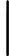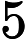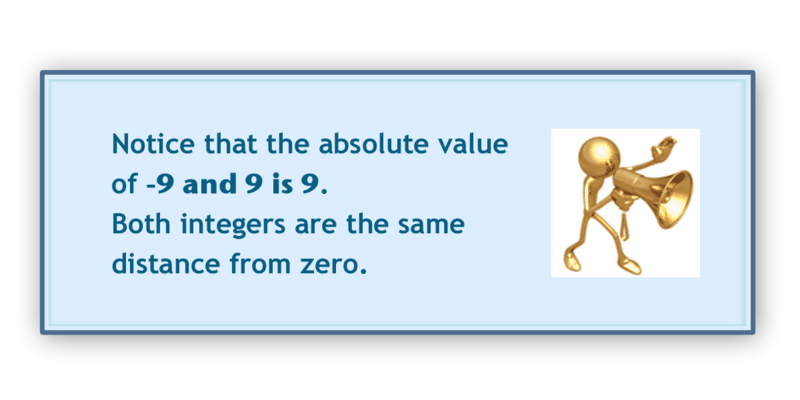Unit 2 Math Absolute Value

Absolute Value Video

Absolute Value On Number Line Video

Absolute Value QUIZIZZ     Game Code: 357515

Vocabulary

Integer
the set of whole numbers and their opposites. Positive and negative whole numbers are integers.
Absolute Value
the number of units that an integer is from zero. The sign does not make a difference.

Have you ever studied mountain ranges? Take a look at this dilemma.

When Kelly came home from school, she began to do a little research on mountain ranges. She found that the city of La Quinta in California is 56 feet above sea level and that the Santa Rosa Mountains are nestled around it.

"Very interesting," Kelly thought to herself.

After reading about mountains, Kelly spotted a book that her brother had on scuba diving. She spotted a picture of a queen angel fish that was taken at a depth of 56 feet.

"Wow, 56 again," Kelly said aloud.

If sea level is 0 and the surface of the water is considered 0, then these two items are the same distance from zero. Here is how we can write them.

+56

-56

They have the same distance from zero, and this is an important thing in mathematics.

Pay attention and you will see why through the information in this Concept.

Guidance

Using a number line allows you the opportunity to see where a number is in relationship to other numbers. We can also see how far a number is from zero.If you look at this number line, you will see that 2 is two units away from zero. We call each little line on the number line a unit. You will also see that -2 is two units away from zero. Even though these numbers have completely different values, one is a loss and the other is a gain, they are both two units away from zero.

The absolute value of a number is the distance that the integer is from zero.

When you want to find the absolute value of a number, the distance the number is from zero, you will see this symbol around the number. The absolute value of 5 is 5. Five is five units away from zero.

The absolute value of negative nine is the number of units that -9 is from zero. It is nine units from zero. So the absolute value of -9 is 9.